Next: Scattering in the Center Up: Two-Body Dynamics Previous: Reduced Mass

Binary Star Systems

Approximately half of the stars in our galaxy are members of so-called binary star systems. Such systems consist of two stars orbiting about their common center of mass. The distance separating the stars is always much less than the distance to the nearest neighbour star. Hence, a binary star system can be treated as a two-body dynamical system to a very good approximation.

In a binary star system, the gravitational force which the first star exerts on the second is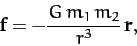(329)

where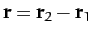. As we have seen, a two-body system can be reduced to an equivalent one-body system whose equation of motion is of the form (327), where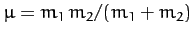. Hence, in this particular case, we can write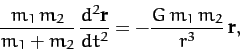(330)

which gives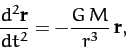(331)

where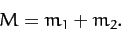(332)

Equation (331) is identical to Equation (212), which we have already solved. Hence, we can immediately write down the solution: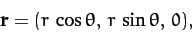(333)

where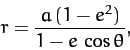(334)

and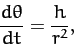(335)

with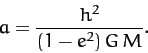(336)

Here,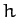is a constant, and we have aligned our Cartesian axes so that the plane of the orbit coincides with the-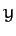plane. According to the above solution, the second star executes a Keplerian elliptical orbit, with major radius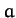and eccentricity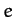, relative to the first star, and vice versa. From Equation (258), the period of revolution,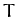, is given by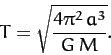(337)

In the inertial frame of reference whose origin always coincides with the center of mass--the so-called center of mass frame--the position vectors of the two stars are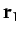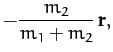(338)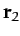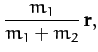(339)

where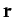is specified above. Figure 20 shows an example binary star orbit, in the center of mass frame, calculated with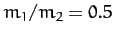and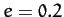. Here, the triangles and squares denote the positions of the first and second star, respectively (which are always diagrammatically opposite one another, as indicated by the arrows). It can be seen that both stars execute elliptical orbits about their common center of mass.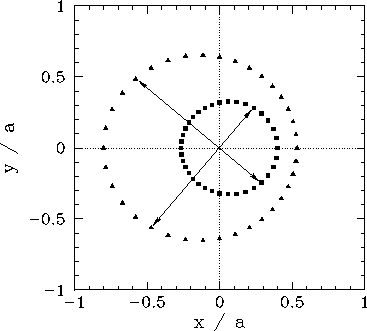Binary star systems have been very useful to astronomers, since it is possible to determine the masses of both stars in such a system by careful observation. The sum of the masses of the two stars,, can be found from Equation (337) after a measurement of the major radius,(which is the mean of the greatest and smallest distance apart of the two stars during their orbit), and the orbital period,. The ratio of the masses of the two stars,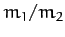, can be determined from Equations (338) and (339) by observing the fixed ratio of the relative distances of the two stars from the common center of mass about which they both appear to rotate. Obviously, given the sum of the masses, and the ratio of the masses, the individual masses themselves can then be calculated.Next: Scattering in the Center Up: Two-Body Dynamics Previous: Reduced Mass
Richard Fitzpatrick 2011-03-31